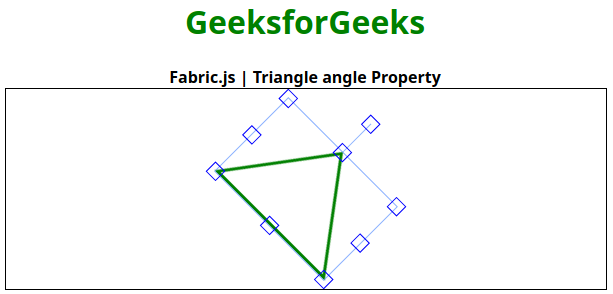Fabric.js | Triangle angle Property

• Last Updated : 02 Feb, 2021

In this article, we are going to see how to draw a fixed angled canvas Triangle using FabricJS. The canvas triangle means the triangle is movable and can be stretched according to requirement. Further, the triangle can be customized when it comes to initial stroke color, height, width, fill color, or stroke width.

To make this possible we are going to use a JavaScript library called FabricJS. After importing the library, we will create a canvas block in the body tag which will contain the triangle. After this, we will initialize instances of Canvas and triangle provided by FabricJS and enable the angle of the canvas triangle using angle property and render the triangle on the Canvas as given below.

Syntax:

fabric.Triangle({
width: number,
height: number,
angle: number
});

Parameters: This function accepts three parameters as mentioned above and described below:

• width: It specifies the width of triangle.
• height: It specifies the height of triangle.
• angle: This parameter defines the angle of the triangle.

Example: This example uses FabricJS to enable the angle of the canvas-like triangle as given below. Try angle the object after enabling angle property, it will rotate the triangle by defined number.

Example:

HTML

              Fabric.js | Triangle angle Property

Output:My Personal Notes arrow_drop_up